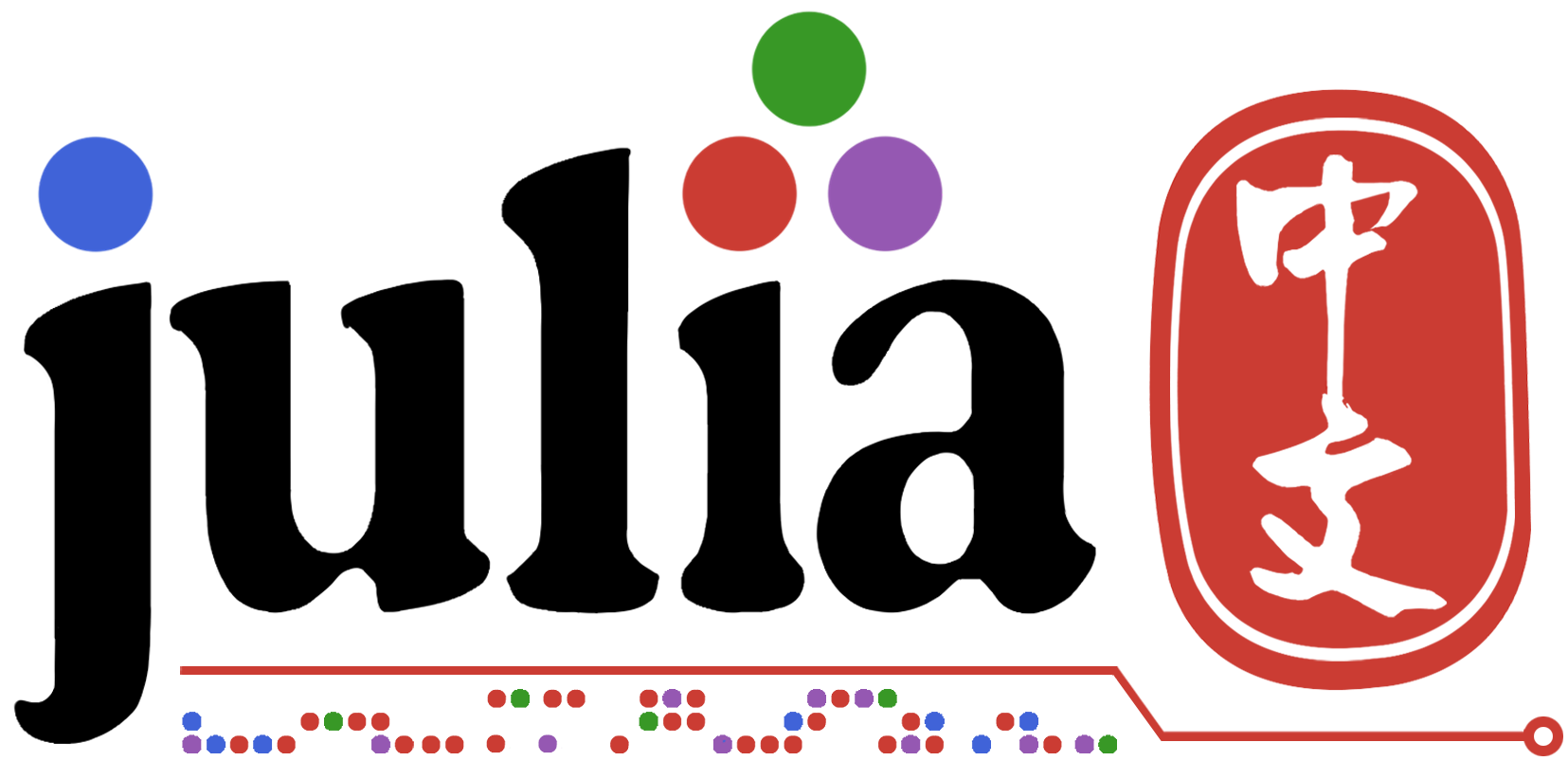# 同一数据类型，hash结果却不一样！？

``````julia> t1.term
Inheritance(Product(Term[Word("a"), Word("b")]), Word("b"))

Inheritance(Product(Term[Word("a"), Word("b")]), Word("b"))

false
``````

``````abstract type Term end
abstract type Compound <: Term end
abstract type Statement <: Term end

struct Product <: Compound
comps::Vector{Term}
end

struct Word <: Term
literal::String
end

struct Inheritance <: Statement
t1::Term
t2::Term
end
``````

``````julia> a1 = Inheritance(Product(Term[Word("a"), Word("b")]), Word("b"))
Inheritance(Product(Term[Word("a"), Word("b")]), Word("b"))

julia> a2 = deepcopy(a1)
Inheritance(Product(Term[Word("a"), Word("b")]), Word("b"))

julia> hash(a1)
0xcb656deaa853ba50

julia> hash(a2)
0xff771b2c3c5eab2d
``````

``````julia> hash([1,2])
0x8fd27f36c41781c8
``````

``````Base.hash(cpd::Compound, h::UInt) = hash(cpd.comps, h)
Base.hash(st::Statement, h::UInt) = hash(st.t1, hash(st.t2, h))
``````

``````New types should implement the 2-argument form, typically by calling the 2-argument `hash`
method recursively in order to mix hashes of the contents with each other (and with `h`).
Typically, any type that implements `hash` should also implement its own `==` (hence
`isequal`) to guarantee the property mentioned above. Types supporting subtraction
(operator `-`) should also implement [`widen`](@ref), which is required to hash
values inside heterogeneous arrays.
``````

``````import Base.hash
function hash(x::Product)
hash(x.comps)
end
function hash(x::Inheritance)
hash(x.t1) ⊻ hash(x.t2)
end

julia> a1 = Inheritance(Product(Term[Word("a"), Word("b")]), Word("b"))
Inheritance(Product(Term[Word("a"), Word("b")]), Word("b"))

julia> a2 = deepcopy(a1)
Inheritance(Product(Term[Word("a"), Word("b")]), Word("b"))

julia> hash(a1)
0xa858c484eaa939fb

julia> hash(a2)
0xa858c484eaa939fb
``````

1赞